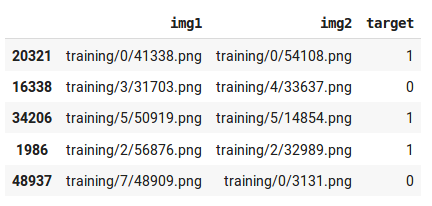# Siamese Network using custom classes

I am trying to implement Siamese Network on MNIST dataset in fastai v3 using contrastive loss not triplet loss. This network will take two images as input and target variable will be 1 or 0 depending whether they belong to same class or different.

I already have dataframe which contains pairs of images and targets.There are two different ways to create databunch.

1. First way is to create custom ItemBase and ItemList to read two images as described by Alex Fitts on Humpback Whales Challenge.

Custom ItemBase

``````mean, std = torch.tensor(mnist_stats)

# The primary difference from the tutorial is with how normalization is being done here
class SiamImage(ItemBase):
def __init__(self, img1, img2): ## These should of Image type
self.img1, self.img2 = img1, img2
self.obj, self.data = (img1, img2), [(img1.data-mean[...,None,None])/std[...,None,None], (img2.data-mean[...,None,None])/std[...,None,None]]
def apply_tfms(self, tfms,*args, **kwargs):
self.img1 = self.img1.apply_tfms(tfms, *args, **kwargs)
self.img2 = self.img2.apply_tfms(tfms, *args, **kwargs)
self.data = [(self.img1.data-mean[...,None,None])/std[...,None,None], (self.img2.data-mean[...,None,None])/std[...,None,None]]
return self
def __repr__(self): return f'{self.__class__.__name__} {self.img1.shape, self.img2.shape}'
def to_one(self):
return Image(mean[...,None,None]+torch.cat(self.data,2)*std[...,None,None])

``````

Custom ItemList

``````class SiamImageItemList(ImageItemList):
def __init__(self, *args, **kwargs):
super().__init__(*args, **kwargs)
#         self._label_cls=FloatList

def __len__(self)->int: return len(self.items) or 1

def get(self, i):
match=1
if i>=len(self.items)//2:#"First set of iteration will generate similar pairs, next will generate different pairs"
match = 0
fn = self.items[i]
img1 = super().get(i) # Returns Image class object

imgs = self.xtra.Image.values
ids = self.xtra.Id.values
wcls = ids[i]
simgs = imgs[ids == wcls]
dimgs = imgs[ids != wcls]
if len(simgs)==1 and match==1:
fn2=fn
else:
while True:
np.random.shuffle(simgs)
np.random.shuffle(dimgs)
if simgs != fn:
fn2 = [simgs if match==1 else dimgs]
break
fn2 = self.items[np.where(imgs==fn2)]
img2 = super().open(fn2) # Returns Image class object
return SiamImage(img1, img2)

def reconstruct(self, t): return SiamImage(mean[...,None,None]+t*std[...,None,None], mean[...,None,None]+t*std[...,None,None])

def show_xys(self, xs, ys, figsize:Tuple[int,int]=(9,10), **kwargs):
rows = int(math.sqrt(len(xs)))
fig, axs = plt.subplots(rows,rows,figsize=figsize)
for i, ax in enumerate(axs.flatten() if rows > 1 else [axs]):
xs[i].to_one().show(ax=ax, y=ys[i], **kwargs)
plt.tight_layout()
``````
1. Second way is to create custom DataBunch as shown by radek.

Custom DataBunch

``````def is_even(num): return num % 2 == 0

class TwoImDataset(Dataset):
def __init__(self, ds):
self.ds = ds
self.whale_ids = ds.y.items
def __len__(self):
return 2 * len(self.ds)
def __getitem__(self, idx):
if is_even(idx):
return self.sample_same(idx // 2)
else: return self.sample_different((idx-1) // 2)
def sample_same(self, idx):
whale_id = self.whale_ids[idx]
candidates = list(np.where(self.whale_ids == whale_id))
candidates.remove(idx) # dropping our current whale - we don't want to compare against an identical image!

if len(candidates) == 0: # oops, there is only a single whale with this id in the dataset
return self.sample_different(idx)

np.random.shuffle(candidates)
return self.construct_example(self.ds[idx], self.ds[candidates], 1)
def sample_different(self, idx):
whale_id = self.whale_ids[idx]
candidates = list(np.where(self.whale_ids != whale_id))
np.random.shuffle(candidates)
return self.construct_example(self.ds[idx], self.ds[candidates], 0)

def construct_example(self, im_A, im_B, class_idx):
return [im_A, im_B], class_idx
``````

My question is,
Is there any way to use first column and second column of the dataframe to create databunch `from_df(df, path, cols=['img_1', 'img_2'])` directly?

As both the approaches require writing the code for creating similar and different image pairs, I already have the dataframe of similar and different image pairs along with targets. (why implement it again)

``````data = (ImageList.from_df(new_df, path, cols=['img1', 'img2'])
.split_by_rand_pct(0.2, seed=42)
.label_from_df(cols=['target'])
.transforms(tfms, size=28)
.databunch(bs=bs))
``````

Is there any way to write custom `get() or from_df()` function to consider the first column as one items and second columns as another items?

I’ve had some progress with generating a dataset for Siamese Networks:

Hello dudeperf3ct, did you find some way to custom get() or from_df() to read already prepared image couples from dataframe (with prepared target = 0 or 1) ?

Hi,

I do have the same problem and I don’t know how to fix it.
Have you found a solution for this?

I solved that problem. I could able to train from dataframe directly.